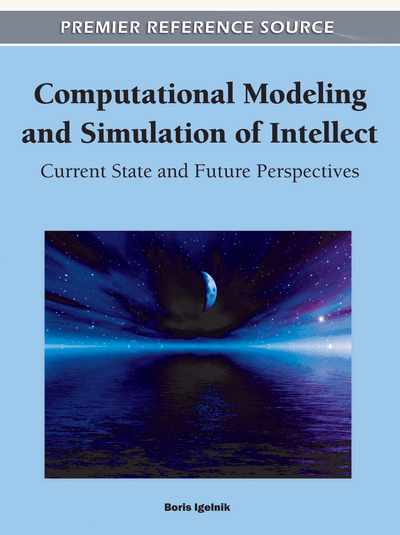# The Kolmogorov Spline Network for Image Processing

Pierre-Emmanuel Leni (University of Burgundy, France), Yohan D. Fougerolle (University of Burgundy, France) and Frédéric Truchetet (University of Burgundy, France)
DOI: 10.4018/978-1-60960-551-3.ch002
Available
\$37.50
No Current Special Offers

## Abstract

In 1900, Hilbert stated that high order equations cannot be solved by sums and compositions of bivariate functions. In 1957, Kolmogorov proved this hypothesis wrong and presented his superposition theorem (KST) that allowed for writing every multivariate functions as sums and compositions of univariate functions. Sprecher has proposed in (Sprecher, 1996) and (Sprecher, 1997) an algorithm for exact univariate function reconstruction. Sprecher explicitly describes construction methods for univariate functions and introduces fundamental notions for the theorem comprehension (such as tilage). Köppen has presented applications of this algorithm to image processing in (Köppen, 2002) and (Köppen & Yoshida, 2005). The lack of flexibility of this scheme has been pointed out and another solution which approximates the univariate functions has been considered. More specifically, it has led us to consider Igelnik and Parikh’s approach, known as the KSN which offers several perspectives of modification of the univariate functions as well as their construction. This chapter will focus on the presentation of Igelnik and Parikh’s Kolmogorov Spline Network (KSN) for image processing and detail two applications: image compression and progressive transmission. Precisely, the developments presented in this chapter include: (1)Compression: the authors study the reconstruction quality using univariate functions containing only a fraction of the original image pixels. To improve the reconstruction quality, they apply this decomposition on images of details obtained by wavelet decomposition. The authors combine this approach into the JPEG 2000 encoder, and show that the obtained results improve JPEG 2000 compression scheme, even at low bitrates. (2)Progressive Transmission: the authors propose to modify the generation of the KSN. The image is decomposed into univariate functions that can be transmitted one after the other to add new data to the previously transmitted functions, which allows to progressively and exactly reconstruct the original image. They evaluate the transmission robustness and provide the results of the simulation of a transmission over packet-loss channels.
Chapter Preview
Top

## Introduction

The Superposition Theorem is the solution to one of the 23 mathematical problems conjectured by Hilbert in 1900. Hilbert suggested that solutions to high order equations were at least bivariate functions. This hypothesis was proven wrong by Kolmogorov in 1957 when he showed that continuous multivariate functions can be expressed as sums and compositions of univariate functions as

(1)

Our goal is to investigate some aspects of this decomposition for images by considering a gray level discrete image as a sample of a continuous bivariate function. To achieve such decomposition, the univariate functionsandmust be constructed. The functionsare called outer or external functions, and the functionsare called inner or internal functions.

A construction method has been proposed in (Sprecher, 1996) and (Sprecher, 1997). Sprecher provides an explicit and exact computation algorithm for the values of the univariate functions at a user defined precision. The Kolmogorov superposition theorem, as reformulated and simplified by Sprecher, can be written as

(2)

We have implemented Sprecher’s algorithm, using the improvements proposed in (Braun and Griebel, 2007) to guarantee the monotonicity of the internal function, and tested it on image decomposition in (Leni et al., 2008). In this algorithm, the univariate functions can be exactly computed for any coordinates of the definition space of the multivariate function at a given precision, but no analytic expression exists. One of the main drawbacks of this approach is that the univariate function definitions cannot be modified without completely modifying the overall pipeline of this method. This issue has led us to consider Igelnik and Parikh’s approach, known as Kolmogorov Spline Network (KSN), that approximates the univariate functions as well as offers a greater flexibility on several parameters of the algorithm (i.e., univariate function constructions, number of layers, tile size, and so on).

We present in detail the original KSN introduced by Igelnik and Parikh, then we propose some modifications for two compression and progressive transmission.

In the KSN, a multivariate function f is approximated by

(3)

## Complete Chapter List

Search this Book:
Reset